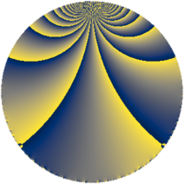# Properties

 Label 441.4.bdLevel $441$ Weight $4$ Character orbit 441.bd Rep. character $\chi_{441}(47,\cdot)$ Character field $\Q(\zeta_{42})$ Dimension $1992$ Sturm bound $224$

# Related objects

## Defining parameters

 Level: $$N$$ $$=$$ $$441 = 3^{2} \cdot 7^{2}$$ Weight: $$k$$ $$=$$ $$4$$ Character orbit: $$[\chi]$$ $$=$$ 441.bd (of order $$42$$ and degree $$12$$) Character conductor: $$\operatorname{cond}(\chi)$$ $$=$$ $$441$$ Character field: $$\Q(\zeta_{42})$$ Sturm bound: $$224$$

## Dimensions

The following table gives the dimensions of various subspaces of $$M_{4}(441, [\chi])$$.

Total New Old
Modular forms 2040 2040 0
Cusp forms 1992 1992 0
Eisenstein series 48 48 0

## Trace form

 $$1992q - 18q^{2} - 11q^{3} - 664q^{4} - 15q^{5} + 38q^{6} - 12q^{7} + 169q^{9} + O(q^{10})$$ $$1992q - 18q^{2} - 11q^{3} - 664q^{4} - 15q^{5} + 38q^{6} - 12q^{7} + 169q^{9} - 22q^{10} - 21q^{11} - 396q^{12} - 43q^{13} - 150q^{14} + 59q^{15} + 2560q^{16} - 72q^{17} + 24q^{18} - 36q^{19} + 3q^{20} + 292q^{21} - 21q^{22} + 861q^{23} + 52q^{24} - 7905q^{25} - 96q^{26} + 376q^{27} + 128q^{28} - 771q^{29} - 7q^{30} - 198q^{31} - 210q^{32} - 863q^{33} + 17q^{34} + 765q^{35} + 298q^{36} + 1066q^{37} + 1725q^{38} + 531q^{39} - 7q^{40} + 597q^{41} + 5261q^{42} - 89q^{43} + 795q^{44} + 1447q^{45} + 3234q^{46} + 180q^{47} - 1569q^{48} - 522q^{49} + 675q^{50} - 263q^{51} - 1351q^{52} + 1092q^{53} + 670q^{54} - 2170q^{55} - 3621q^{56} + 1871q^{57} - 1233q^{58} - 768q^{59} - 6912q^{60} - 4678q^{61} - 3072q^{62} - 1571q^{63} + 19548q^{64} - 1194q^{65} - 525q^{66} - 288q^{67} - 7050q^{68} - 6479q^{69} + 383q^{70} + 9702q^{71} - 315q^{72} - 22q^{73} - 21q^{74} - 2384q^{75} + 297q^{76} + 1182q^{77} + 8991q^{78} + 90q^{79} - 4239q^{80} + 7505q^{81} - 46q^{82} + 1809q^{83} + 23489q^{84} - 130q^{85} - 21q^{86} + 1388q^{87} - 1253q^{88} - 10014q^{89} + 7585q^{90} + 1112q^{91} + 21885q^{92} - 13700q^{93} - 4q^{94} - 3657q^{95} + 391q^{96} - 792q^{97} + 5667q^{98} + 9845q^{99} + O(q^{100})$$

## Decomposition of $$S_{4}^{\mathrm{new}}(441, [\chi])$$ into newform subspaces

The newforms in this space have not yet been added to the LMFDB.